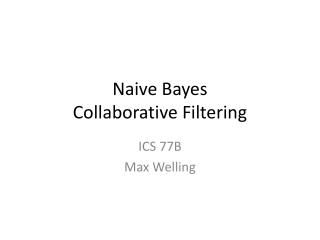DownloadDownload PresentationNaive Bayes Collaborative Filtering

# Naive Bayes Collaborative Filtering

Download Presentation## Naive Bayes Collaborative Filtering

- - - - - - - - - - - - - - - - - - - - - - - - - - - E N D - - - - - - - - - - - - - - - - - - - - - - - - - - -
##### Presentation Transcript

1. Naive Bayes Collaborative Filtering ICS 77B Max Welling

2. We want to compute the probability that: • Item 5 will be rated 1, given that • Item 1 was rated 1 and • Item 2 was rated 3 and • Item 3 was rated 3, …. • Item 5 will be rated 2 given that • Item 1 was rated 1 and • Item 2 was rated 3, …

3. To compute these probabilities we first ask: “how frequently did other users rate R1 = 1 & R5 = 1 ? We see that User 2 and User 4 both rated R1 = 1 & R5 = 1. That’s 100% ! Question: What is the probability that R1 = 2 | R5 = 4 ? In the end we will have computed all P(R1=x1|R5=y), P(R2=x2|R5=y), P(R3=x3|R5=y), P(R4=x4|R5=y)

4. We can also compute: P(R5 = 1), P(R5 = 2), P(R5 = 3), P(R5 = 4), P(R5 = 5). Question: Compute probability that: P(R5 = 1).

5. In the end we thus have: P(Ri=xi|R5 = y) for i=1,2,3,4 P(R5 = y) How do we combine this: Bayes rule! P(y|x) P(x) = P(x|y) P(y)  P(y|x) = P(x|y) P(y) / P(x)

6. P(y|x) P(x) = P(x|y) P(y)  P(y|x) = P(x|y) P(y) / P(x) We will also use that we assume the rating for different items to be conditionally independent: P(R1=x1,R2=x2,R3=x3,R4=x4|R5=y) = P(R1=x1|R5=y) P(R2=x2|R5=y) P(R3=x3|R5=y) P(R4=x4|R5=y)

7. Combining: P(R5=y|R1=1,R2=3,R3=3,R4=2) = P(R1=x1|R5=y) P(R2=x2|R5=y) P(R3=x3|R5=y) P(R4=x4|R5=y) P(R5=y) / constant  Try all values of y and pick the one which has largest probability.